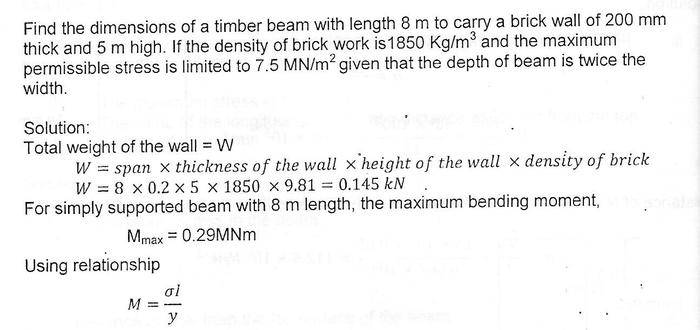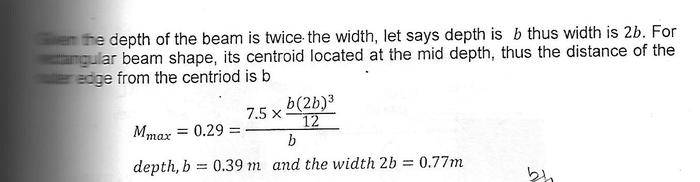# Maximum bending moment

## Homework Statement

how the author found the maximum moment = 0.29MNm ? is there any formula ? how to find it in this question ?SteamKing
Staff Emeritus
Homework Helper

## Homework Statement

how the author found the maximum moment = 0.29MNm ? is there any formula ? how to find it in this question ?## The Attempt at a Solution

Of course there's a formula. That's what beam tables are for - too look up things like the maximum B.M. without having to work them out from scratch all the time.

Here is a set of typical beam tables:

http://www.awc.org/pdf/codes-standards/publications/design-aids/AWC-DA6-BeamFormulas-0710.pdf

Your beam is Figure 1, page 4.

BTW, the calculation of the total weight of the wall above has a small error. W = 0.145 MN, rather than 0.145 kN.

Of course there's a formula. That's what beam tables are for - too look up things like the maximum B.M. without having to work them out from scratch all the time.

Here is a set of typical beam tables:

http://www.awc.org/pdf/codes-standards/publications/design-aids/AWC-DA6-BeamFormulas-0710.pdf

Your beam is Figure 1, page 4.

BTW, the calculation of the total weight of the wall above has a small error. W = 0.145 MN, rather than 0.145 kN.
the shape is rectangular cross section , am i right ? why the moment shouldnt' be a(b^3) / 12 ? but , a(b^2) / 8 ?

SteamKing
Staff Emeritus